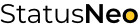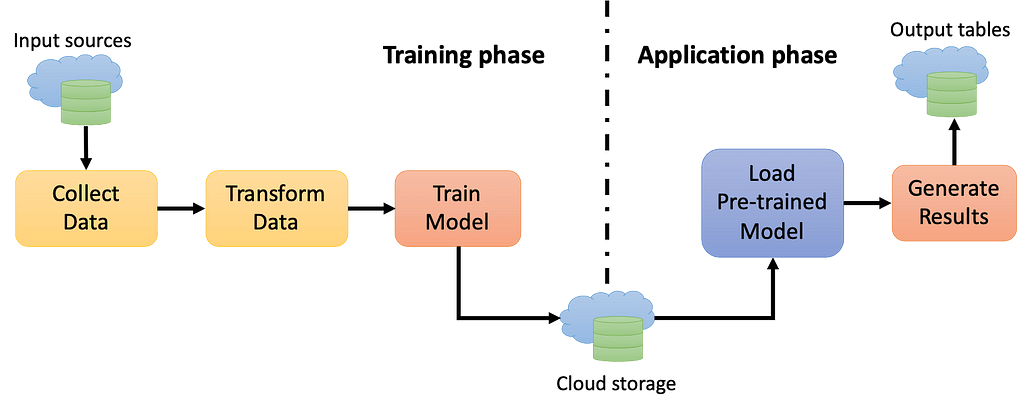WE ARE EXPERTS IN TECHNOLOGY

## Let’s Work Together# StatusNeo## Building a Machine Learning (ML) Model with PySpark

Introduction

Machine learning (ML) is a subset of artificial intelligence (AI) that enables machines to learn from data and improve their performance over time. Building an ML model involves several steps, including data preparation, feature engineering, model selection, and evaluation. In this paper, we will discuss how to build an ML model using PySpark, a distributed computing framework for big data processing. We will cover the key steps involved in building an ML model with PySpark and provide examples of how to perform each step.

Data Preparation

The first step in building an ML model is to prepare the data. This involves loading the data into PySpark and performing some initial data cleaning and preprocessing. PySpark provides several libraries for loading and manipulating data, including the DataFrame API and the RDD API.

Let’s assume we have a dataset that contains information about customers’ purchases, such as the product ID, the customer ID, and the purchase date. We can load this dataset into PySpark using the DataFrame API as follows:

python
from pyspark.sql import SparkSession
spark = SparkSession.builder.appName(“Purchases”).getOrCreate()

This code reads the CSV file “purchases.csv” and creates a DataFrame object called “df”. The “header=True” parameter indicates that the first row of the CSV file contains the column names, and the “inferSchema=True” parameter tells PySpark to infer the data types of each column.

Once we have loaded the data, we can perform some initial data cleaning and preprocessing. For example, we can remove any rows with missing data using the “dropna” method:

python
df = df.dropna()
Feature Engineering

The next step is to perform feature engineering, which involves selecting the relevant features from the dataset and transforming them into a format suitable for the ML model. Feature engineering is a critical step in building an ML model, as it directly impacts the model’s performance.

In our example, let’s assume we want to predict whether a customer will purchase a product again within a certain period. We can extract the relevant features from the dataset, such as the customer’s purchase history and the product’s popularity. We can then transform these features into a format suitable for the ML model, such as a vector.

python
from pyspark.ml.feature import VectorAssembler
assembler = VectorAssembler(inputCols=[“customer_id”, “product_id”, “product_popularity”], outputCol=”features”)
data = assembler.transform(df)
This code creates a VectorAssembler object that combines the “customer_id”, “product_id”, and “product_popularity” columns into a vector called “features”. The “transform” method applies the transformation to the DataFrame “df” and creates a new DataFrame called “data”.

Model Selection and Training

The next step is to select an appropriate ML model and train it on the transformed data. PySpark provides several ML libraries, including the MLlib library, which contains a range of algorithms for classification, regression, and clustering.

Let’s assume we want to use logistic regression to predict whether a customer will purchase a product again within a certain period. We can create a logistic regression model using the LogisticRegression class and train it on the transformed data.

python
from pyspark.ml.classification import LogisticRegression
lr = LogisticRegression(featuresCol=”features”, labelCol=”label”)
model = lr.fit(data)
This code creates a LogisticRegression object with the “features” vector as the input and the “label” column as the output. The “fit” method trains the model on the transformed data and creates a new model object called “model”.

Model Evaluation

The final step is to evaluate the performance of the trained model.

Spark provides several evaluation metrics for classification, such as accuracy, precision, recall, and F1-score. We can use these metrics to evaluate the performance of our logistic regression model.

```python
```from pyspark.ml.evaluation import BinaryClassificationEvaluator
predictions = model.transform(data)
evaluator = BinaryClassificationEvaluator(rawPredictionCol="rawPrediction", labelCol="label")
accuracy = evaluator.evaluate(predictions, {evaluator.metricName: "accuracy"})
precision = evaluator.evaluate(predictions, {evaluator.metricName: "precision"})
recall = evaluator.evaluate(predictions, {evaluator.metricName: "recall"})
f1_score = evaluator.evaluate(predictions, {evaluator.metricName: "f1"})
print("Accuracy:", accuracy)
print("Precision:", precision)
print("Recall:", recall)
print("F1-score:", f1_score)
``````

This code applies the trained model to the transformed data and generates predictions. The BinaryClassificationEvaluator class is used to evaluate the performance of the model. We can calculate several evaluation metrics, including accuracy, precision, recall, and F1-score.

Conclusion

Building an ML model with PySpark involves several steps, including data preparation, feature engineering, model selection, and evaluation. PySpark provides several libraries for loading and manipulating data, selecting features, and training ML models. The MLlib library contains a range of algorithms for classification, regression, and clustering. Once we have trained the model, we can use PySpark’s evaluation metrics to evaluate its performance. Building an ML model with PySpark can be challenging, but with the right approach, it can be a powerful tool for analyzing large datasets and extracting insights.